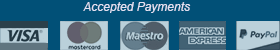﻿ Mathematical Word Problems - Clerical Ability - Aptitude-Test.com

# Clerical Ability - Mathematical Word Problems

Mathematical word problems, are simple mathematical questions presented in a verbal format. The questions will assess your mathematical ability. Because of the nature of mathematical word problems, calculators are not allowed. However, pen and paper is almost always provided.

Unlike numerical word problems, the word problems in clerical ability tests are not logical reasoning questions.

The mathematical word problems will most commonly be a simple mathematical equation presented in a verbal format. Please see the example below:

Question
Oliver took 56 marbles and divided them into 8 equal groups. How many marbles were there in each group?

A) 8
B) 6
C) 7
D) 5